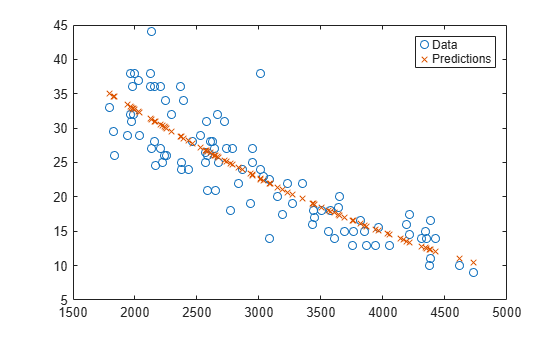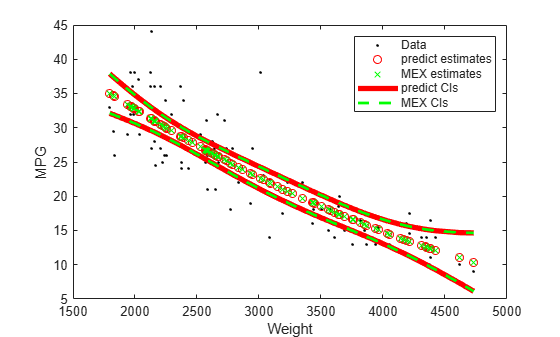# predict

Predict responses of linear regression model

## Syntax

``ypred = predict(mdl,Xnew)``
``````[ypred,yci] = predict(mdl,Xnew)``````
``````[ypred,yci] = predict(mdl,Xnew,Name,Value)``````

## Description

example

````ypred = predict(mdl,Xnew)` returns the predicted response values of the linear regression model `mdl` to the points in `Xnew`.```
``````[ypred,yci] = predict(mdl,Xnew)``` also returns confidence intervals for the responses at `Xnew`.```

example

``````[ypred,yci] = predict(mdl,Xnew,Name,Value)``` specifies additional options using one or more name-value pair arguments. For example, you can specify the confidence level of the confidence interval and the prediction type.```

## Examples

collapse all

Create a quadratic model of car mileage as a function of weight from the `carsmall` data set.

```load carsmall X = Weight; y = MPG; mdl = fitlm(X,y,'quadratic');```

Create predicted responses to the data.

`ypred = predict(mdl,X);`

Plot the original responses and the predicted responses to see how they differ.

```plot(X,y,'o',X,ypred,'x') legend('Data','Predictions')```Fit a linear regression model, and then save the model by using `saveLearnerForCoder`. Define an entry-point function that loads the model by using `loadLearnerForCoder` and calls the `predict` function of the fitted model. Then use `codegen` (MATLAB Coder) to generate C/C++ code. Note that generating C/C++ code requires MATLAB® Coder™.

This example briefly explains the code generation workflow for the prediction of linear regression models at the command line. For more details, see Code Generation for Prediction of Machine Learning Model at Command Line. You can also generate code using the MATLAB Coder app. For details, see Code Generation for Prediction of Machine Learning Model Using MATLAB Coder App.

Train Model

Load the `carsmall` data set, and then fit the quadratic regression model.

```load carsmall X = Weight; y = MPG; mdl = fitlm(X,y,'quadratic');```

Save Model

Save the fitted quadratic model to the file `QLMMdl.mat` by using `saveLearnerForCoder`.

`saveLearnerForCoder(mdl,'QLMMdl');`

Define Entry-Point Function

Define an entry-point function named `mypredictQLM` that does the following:

• Accept measurements corresponding to X and optional, valid name-value pair arguments.

• Load the fitted quadratic model in `QLMMdl.mat`.

• Return predictions and confidence interval bounds.

```function [yhat,ci] = mypredictQLM(x,varargin) %#codegen %MYPREDICTQLM Predict response using linear model % MYPREDICTQLM predicts responses for the n observations in the n-by-1 % vector x using the linear model stored in the MAT-file QLMMdl.mat, and % then returns the predictions in the n-by-1 vector yhat. MYPREDICTQLM % also returns confidence interval bounds for the predictions in the % n-by-2 vector ci. CompactMdl = loadLearnerForCoder('QLMMdl'); [yhat,ci] = predict(CompactMdl,x,varargin{:}); end ```

Add the `%#codegen` compiler directive (or pragma) to the entry-point function after the function signature to indicate that you intend to generate code for the MATLAB algorithm. Adding this directive instructs the MATLAB Code Analyzer to help you diagnose and fix violations that would result in errors during code generation.

Note: If you click the button located in the upper-right section of this example and open the example in MATLAB®, then MATLAB opens the example folder. This folder includes the entry-point function file.

Generate Code

Generate code for the entry-point function using `codegen` (MATLAB Coder). Because C and C++ are statically typed languages, you must determine the properties of all variables in the entry-point function at compile time. To specify the data type and exact input array size, pass a MATLAB® expression that represents the set of values with a certain data type and array size. Use `coder.Constant` (MATLAB Coder) for the names of name-value pair arguments.

If the number of observations is unknown at compile time, you can also specify the input as variable-size by using `coder.typeof` (MATLAB Coder). For details, see Specify Variable-Size Arguments for Code Generation and Specify Properties of Entry-Point Function Inputs (MATLAB Coder).

`codegen mypredictQLM -args {X,coder.Constant('Alpha'),0.1,coder.Constant('Simultaneous'),true}`
```Code generation successful. ```

`codegen` generates the MEX function `mypredictQLM_mex` with a platform-dependent extension.

Verify Generated Code

Compare predictions and confidence intervals using `predict` and `mypredictQLM_mex`. Specify name-value pair arguments in the same order as in the `-args` argument in the call to `codegen`.

```Xnew = sort(X); [yhat1,ci1] = predict(mdl,Xnew,'Alpha',0.1,'Simultaneous',true); [yhat2,ci2] = mypredictQLM_mex(Xnew,'Alpha',0.1,'Simultaneous',true);```

The returned values from `mypredictQLM_mex` might include round-off differences compared to the values from `predict`. In this case, compare the values allowing a small tolerance.

`find(abs(yhat1-yhat2) > 1e-6)`
```ans = 0x1 empty double column vector ```
`find(abs(ci1-ci2) > 1e-6)`
```ans = 0x1 empty double column vector ```

The comparison confirms that the returned values are equal within the tolerance `1e–6`.

Plot the returned values for comparison.

```h1 = plot(X,y,'k.'); hold on h2 = plot(Xnew,yhat1,'ro',Xnew,yhat2,'gx'); h3 = plot(Xnew,ci1,'r-','LineWidth',4); h4 = plot(Xnew,ci2,'g--','LineWidth',2); legend([h1; h2; h3(1); h4(1)], ... {'Data','predict estimates','MEX estimates','predict CIs','MEX CIs'}); xlabel('Weight'); ylabel('MPG');```## Input Arguments

collapse all

Linear regression model object, specified as a `LinearModel` object created by using `fitlm` or `stepwiselm`, or a `CompactLinearModel` object created by using `compact`.

New predictor input values, specified as a table, dataset array, or matrix. Each row of `Xnew` corresponds to one observation, and each column corresponds to one variable.

• If `Xnew` is a table or dataset array, it must contain predictors that have the same predictor names as in the `PredictorNames` property of `mdl`.

• If `Xnew` is a matrix, it must have the same number of variables (columns) in the same order as the predictor input used to create `mdl`. Note that `Xnew` must also contain any predictor variables that are not used as predictors in the fitted model. Also, all variables used in creating `mdl` must be numeric. To treat numerical predictors as categorical, identify the predictors using the `'CategoricalVars'` name-value pair argument when you create `mdl`.

Data Types: `single` | `double` | `table`

### Name-Value Arguments

Specify optional pairs of arguments as `Name1=Value1,...,NameN=ValueN`, where `Name` is the argument name and `Value` is the corresponding value. Name-value arguments must appear after other arguments, but the order of the pairs does not matter.

Before R2021a, use commas to separate each name and value, and enclose `Name` in quotes.

Example: ```[ypred,yci] = predict(Mdl,Xnew,'Alpha',0.01,'Simultaneous',true)``` returns the confidence interval `yci` with a 99% confidence level, computed simultaneously for all predictor values.

Significance level for the confidence interval, specified as the comma-separated pair consisting of `'Alpha'` and a numeric value in the range [0,1]. The confidence level of `yci` is equal to 100(1 – `Alpha`)%. `Alpha` is the probability that the confidence interval does not contain the true value.

Example: `'Alpha',0.01`

Data Types: `single` | `double`

Prediction type, specified as the comma-separated pair consisting of `'Prediction'` and either `'curve'` or `'observation'`.

A regression model for the predictor variables X and the response variable y has the form

y = f(X) + ε,

where f is a fitted regression function and ε is a random noise term.

• If `'Prediction'` is `'curve'`, then `predict` predicts confidence bounds for f(Xnew), the fitted responses at `Xnew`.

• If `'Prediction'` is `'observation'`, then `predict` predicts confidence bounds for y, the response observations at `Xnew`.

The bounds for y are wider than the bounds for f(X) because of the additional variability of the noise term.

Example: `'Prediction','observation'`

Flag to compute simultaneous confidence bounds, specified as the comma-separated pair consisting of `'Simultaneous'` and either true or false.

• `true``predict` computes confidence bounds for the curve of response values corresponding to all predictor values in `Xnew`, using Scheffe's method. The range between the upper and lower bounds contains the curve consisting of true response values with 100(1 – α)% confidence.

• `false``predict` computes confidence bounds for the response value at each observation in `Xnew`. The confidence interval for a response value at a specific predictor value contains the true response value with 100(1 – α)% confidence.

Simultaneous bounds are wider than separate bounds, because requiring the entire curve of response values to be within the bounds is stricter than requiring the response value at a single predictor value to be within the bounds.

Example: `'Simultaneous',true`

## Output Arguments

collapse all

Predicted response values evaluated at `Xnew`, returned as a numeric vector.

Confidence intervals for the responses, returned as a two-column matrix with each row providing one interval. The meaning of the confidence interval depends on the settings of the name-value pair arguments `'Alpha'`, `'Prediction'`, and `'Simultaneous'`.

## Alternative Functionality

• `feval` returns the same predictions as `predict`. The `feval` function can take multiple input arguments, with one input for each predictor variable, which is simpler to use with a model created from a table or dataset array. Note that the `feval` function does not give confidence intervals on its predictions.

• `random` returns predictions with added noise.

• Use `plotSlice` to create a figure containing a series of plots, each representing a slice through the predicted regression surface. Each plot shows the fitted response values as a function of a single predictor variable, with the other predictor variables held constant.

## Version History

Introduced in R2012a